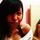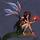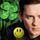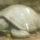## General Question# What is the simple formula for calculating Infiltration Rates?

Asked by IsseyBaby (13) May 4th, 2010

So i have this Geography project regarding the effects of soil types on infiltration rates, and i would like to know the simple formula to calculate the infiltration rate in (mm/min)? Because all the websites that i’ve been to all gave me the formula for (mm/h) :X

Observing members: 0Composing members: 0I would say use the mm/min equation then multiply by 60 to get the mm/hour.

bongo (4297)“Great Answer” (0) Flag as…But what is the mm/min equation?

IsseyBaby (13)“Great Answer” (0) Flag as…If you have the rate in mm/hr you divide that by 60 to get mm/min. (60 minutes in one hour)

LuckyGuy (36376)“Great Answer” (0) Flag as…mm/min = (1/60) * mm/hr

If you make \$60.00 an hour you make \$1.00 a minute.

roundsquare (5512)“Great Answer” (0) Flag as…or